# 1 Introduction

The R package splines2 is intended to be a user-friendly supplementary package to the base package splines. It provides functions to construct a variety of regression spline basis functions that are not available from splines. Most functions have a very similar user interface with the function splines::bs(). More specifically, splines2 allows users to construct the basis functions of

• B-splines
• M-splines
• I-splines
• C-splines
• periodic splines
• natural cubic splines
• generalized Bernstein polynomials

along with their integrals (except C-splines) and derivatives of given order by closed-form recursive formulas.

Compared to splines, the package splines2 provides convenient interfaces for spline derivatives with consistent handling on NA’s. Most of the implementations are in C++ with the help of Rcpp and RcppArmadillo since v0.3.0, which boosted the computational performance.

In the remainder of this vignette, we illustrate the basic usage of most functions in the package through examples. We refer readers to Wang and Yan (2021) for a more formal introduction to the package with applications to shape-restricted regression. See the package manual for more details about function usage.

library(splines2)
packageVersion("splines2")
##  '0.5.1'

# 2 B-splines

## 2.1 B-spline Basis Functions

The bSpline() function generates the basis matrix for B-splines and extends the function bs() of the package splines by providing 1) the piece-wise constant basis functions when degree = 0, 2) the derivatives of basis functions for a positive derivs, 3) the integrals of basis functions if integral = TRUE, 4) periodic basis functions based on B-splines if periodic = TRUE.

One example of linear B-splines with three internal knots is as follows:

knots <- c(0.3, 0.5, 0.6)
x <- seq(0, 1, 0.01)
bsMat <- bSpline(x, knots = knots, degree = 1, intercept = TRUE)
plot(bsMat, mark_knots = "all")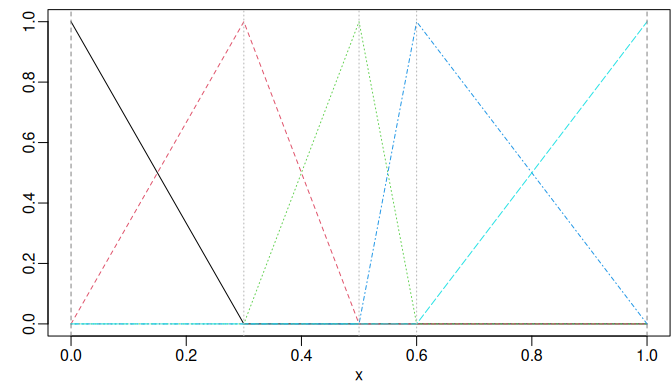B-splines of degree one with three internal knots placed at 0.3, 0.5, and 0.6.

## 2.2 Integrals and Derivatives of B-splines

For convenience, the package also provides functions ibs() and dbs() for constructing the B-spline integrals and derivatives, respectively. Two toy examples are as follows:

ibsMat <- ibs(x, knots = knots, degree = 1, intercept = TRUE)
op <- par(mfrow = c(1, 2))
plot(bsMat, mark_knots = "internal")
plot(ibsMat, mark_knots = "internal")
abline(h = c(0.15, 0.2, 0.25), lty = 2, col = "gray")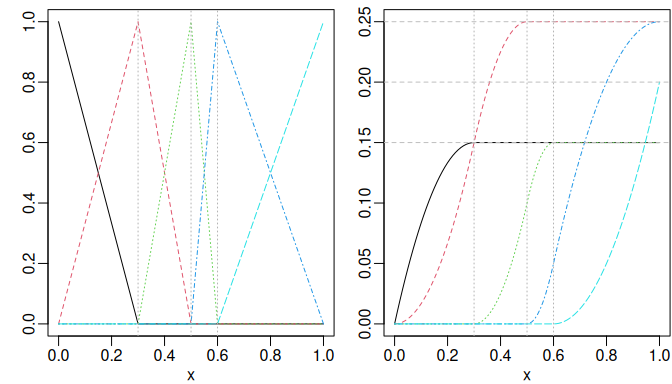Piecewise linear B-splines (left) and their integrals (right).
bsMat <- bSpline(x, knots = knots, intercept = TRUE)
dbsMat <- dbs(x, knots = knots, intercept = TRUE)
plot(bsMat, mark_knots = "internal")
plot(dbsMat, mark_knots = "internal")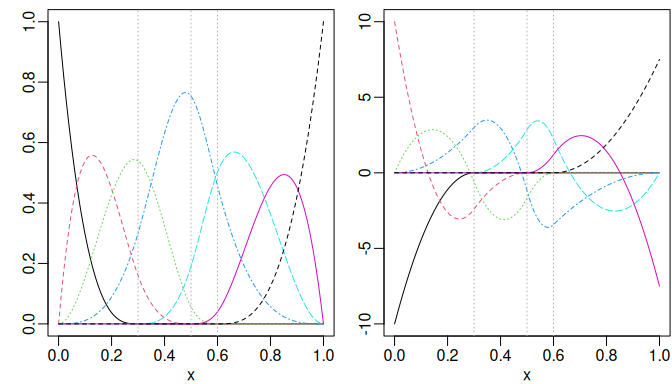Cubic B-spline (left) and their first derivative (right).

We may also obtain the derivatives easily by the deriv() method as follows:

is_equivalent <- function(a, b) {
all.equal(a, b, check.attributes = FALSE)
}
stopifnot(is_equivalent(dbsMat, deriv(bsMat)))

## 2.3 Periodic B-splines

The function bSpline() produces periodic spline basis functions following Piegl and Tiller (1997, chap. 12) when periodic = TRUE is specified. Different from the regular basis functions, the x is allowed to be placed outside the boundary and the Boundary.knots defines the cyclic interval. For instance, one may obtain the periodic cubic B-spline basis functions with cyclic interval (0, 1) as follows:

px <- seq(0, 3, 0.01)
pbsMat <- bSpline(px, knots = knots, Boundary.knots = c(0, 1),
intercept = TRUE, periodic = TRUE)
ipMat <- ibs(px, knots = knots, Boundary.knots = c(0, 1),
intercept = TRUE, periodic = TRUE)
dp1Mat <- deriv(pbsMat)
dp2Mat <- deriv(pbsMat, derivs = 2)
par(mfrow = c(1, 2))
plot(pbsMat, ylab = "Periodic B-splines", mark_knots = "boundary")
plot(ipMat, ylab = "The integrals", mark_knots = "boundary")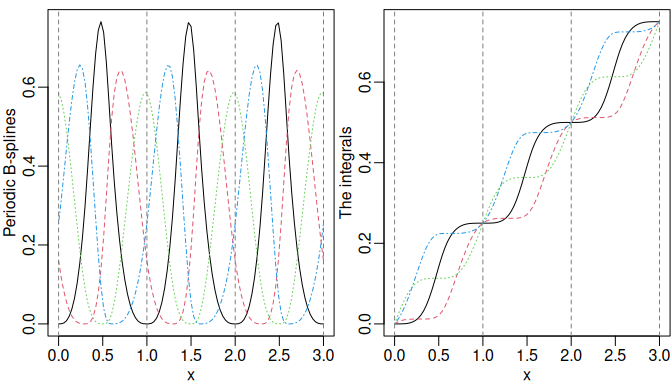plot(dp1Mat, ylab = "The 1st derivatives", mark_knots = "boundary")
plot(dp2Mat, ylab = "The 2nd derivatives", mark_knots = "boundary")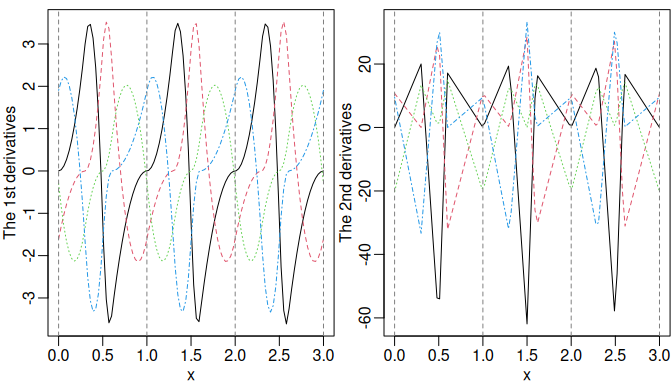For reference, the corresponding integrals and derivatives are also visualized.

# 3 M-Splines

## 3.1 M-spline Basis Functions

M-splines (Ramsay 1988) can be considered the normalized version of B-splines with unit integral within boundary knots. An example given by Ramsay (1988) was a quadratic M-splines with three internal knots placed at 0.3, 0.5, and 0.6. The default boundary knots are the range of x, and thus 0 and 1 in this example.

msMat <- mSpline(x, knots = knots, degree = 2, intercept = TRUE)
par(op)
plot(msMat, mark_knots = "all")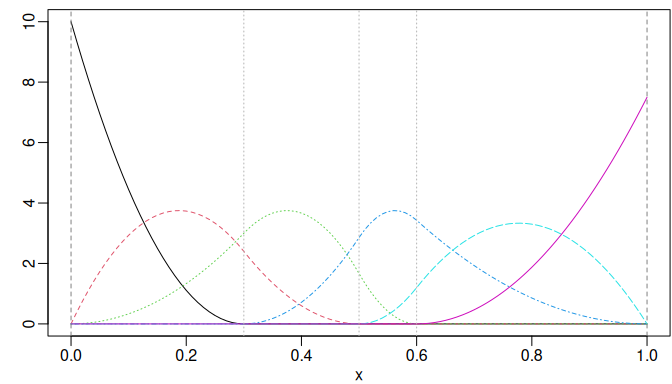Quadratic M-spline with three internal knots placed at 0.3, 0.5, and 0.6.

The derivative of the given order of M-splines can be obtained by specifying a positive integer to argument dervis of mSpline(). Similarly, for an existing mSpline object generated by mSpline(), one can use the deriv() method for derivaitives. For example, the first derivative of the M-splines given in the previous example can be obtained equivalently as follows:

dmsMat1 <- mSpline(x, knots = knots, degree = 2, intercept = TRUE, derivs = 1)
dmsMat2 <- deriv(msMat)
stopifnot(is_equivalent(dmsMat1, dmsMat2))

## 3.2 Periodic M-Splines

The mSpline() function produces periodic splines based on M-spline basis functions when periodic = TRUE is specified. The Boundary.knots defines the cyclic interval, which is the same with the periodic B-splines.

pmsMat <- mSpline(px, knots = knots, intercept = TRUE,
periodic = TRUE, Boundary.knots = c(0, 1))
plot(pmsMat, ylab = "Periodic Basis", mark_knots = "boundary")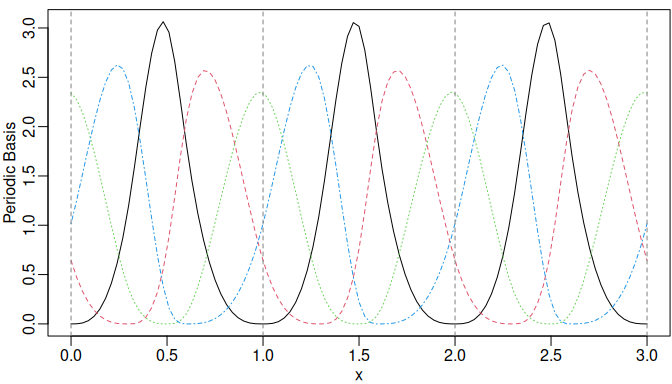Cubic periodic M-splines.

We may still specify the argument derivs in mSpline() or use the corresponding deriv() method to obtain the derivatives when periodic = TRUE.

dpmsMat <- deriv(pmsMat)
plot(dpmsMat, ylab = "The 1st derivatives", mark_knots = "boundary")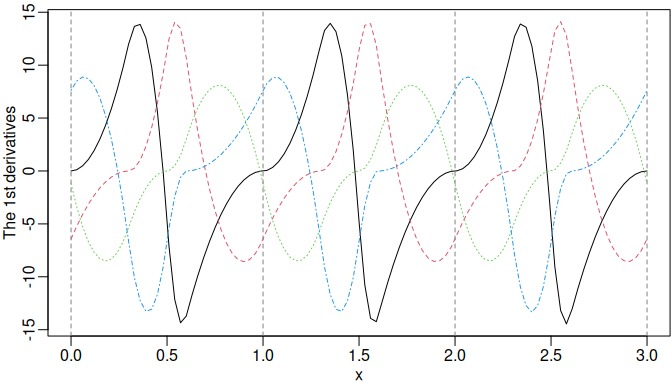The first derivatives of the periodic M-splines.

Furthermore, we can obtain the integrals of the periodic M-splines by specifying integral = TRUE. The integral is integrated from the left boundary knot.

ipmsMat <- mSpline(px, knots = knots, intercept = TRUE,
periodic = TRUE, Boundary.knots = c(0, 1), integral = TRUE)
plot(ipmsMat, ylab = "Integrals", mark_knots = "boundary")
abline(h = seq.int(0, 3), lty = 2, col = "gray")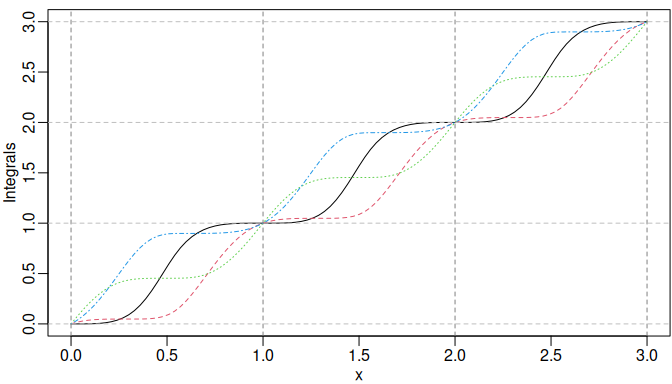The integrals of the periodic M-splines.

# 4 I-Splines

I-splines (Ramsay 1988) are simply the integral of M-splines and thus monotonically nondecreasing with unit maximum value. A monotonically nondecreasing (nonincreasing) function can be fitted by a linear combination of I-spline basis functions with nonnegative (nonpositive) coefficients plus a constant, where the coefficient of the constant is unconstrained.

The example given by Ramsay (1988) was the I-splines corresponding to the quadratic M-splines with three internal knots placed at 0.3, 0.5, and 0.6. Notice that the degree of I-splines is defined from the associated M-splines instead of their polynomial degree.

isMat <- iSpline(x, knots = knots, degree = 2, intercept = TRUE)
plot(isMat, mark_knots = "internal")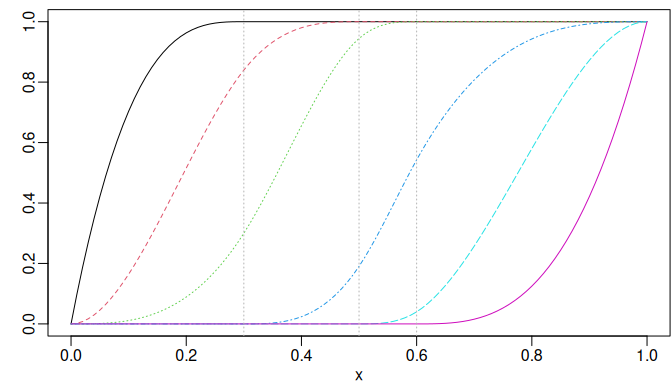I-splines of degree two with three internal knots placed at 0.3, 0.5, and 0.6.

The corresponding M-spline basis matrix can be obtained easily as the first derivatives of the I-splines by the deriv() method.

stopifnot(is_equivalent(msMat, deriv(isMat)))

We may specify derivs = 2 in the deriv() method for the second derivatives of the I-splines, which are equivalent to the first derivatives of the corresponding M-splines.

dmsMat3 <- deriv(isMat, 2)
stopifnot(is_equivalent(dmsMat1, dmsMat3))

# 5 C-Splines

Convex splines (Meyer 2008) called C-splines are scaled integrals of I-splines with unit maximum value at the right boundary knot. Meyer (2008) applied C-splines to shape-restricted regression analysis. The monotone (nondecreasing) property of I-spines ensures the convexity of C-splines. A convex regression function can be estimated using linear combinations of the C-spline basis functions with nonnegative coefficients, plus an unconstrained linear combination of a constant and an identity function $$g(x)=x$$. If the underlying regression function is both increasing and convex, the coefficient on the identity function is restricted to be nonnegative as well.

We may specify the argument scale = FALSE in the function cSpline() to disable the scaling of the integrals of I-splines. Then the actual integrals of the corresponding I-splines will be returned. If scale = TRUE (by default), each C-spline basis is scaled to have unit height at the right boundary knot.

csMat1 <- cSpline(x, knots = knots, degree = 2, intercept = TRUE)
plot(csMat1)
abline(h = 1, v = knots, lty = 2, col = "gray")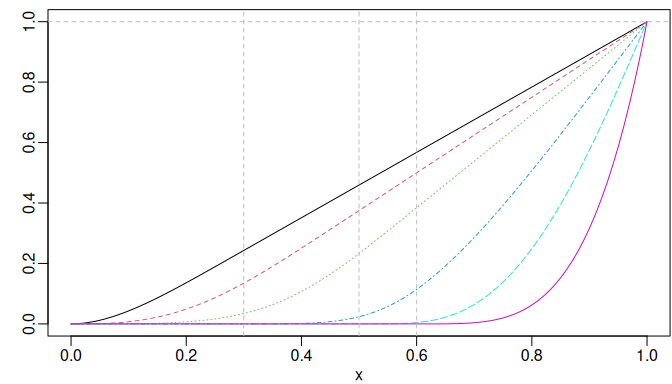C-splines of degree two with three internal knots placed at 0.3, 0.5, and 0.6.

Similarly, the deriv() method can be used to obtain the derivatives. A nested call of deriv() is supported for derivatives of a higher order. However, the argument derivs of the deriv() method can be specified directly for better computational performance. For example, the first and second derivatives can be obtained by the following equivalent approaches, respectively.

csMat2 <- cSpline(x, knots = knots, degree = 2, intercept = TRUE, scale = FALSE)
stopifnot(is_equivalent(isMat, deriv(csMat2)))
stopifnot(is_equivalent(msMat, deriv(csMat2, 2)))
stopifnot(is_equivalent(msMat, deriv(deriv(csMat2))))

# 6 Generalized Bernstein Polynomials

The Bernstein polynomials are equivalent to B-splines without internal knots and have also been applied to shape-constrained regression analysis (e.g., Wang and Ghosh 2012). The $$i$$-th basis of the generalized Bernstein polynomials of degree $$n$$ over $$[a, b]$$ is defined as follows: $B_i^n(x)=\frac{1}{(b-a)^n}{n\choose i}(x-a)^i (b-x)^{n-i},~i\in\{0,\ldots,n\},$ where $$a\le x\le b$$. It reduces to regular Bernstein polynomials defined over $$[0, 1]$$ when $$a = 0$$ and $$b = 1$$.

We may obtain the basis matrix of the generalized using the function bernsteinPoly(). For example, the Bernstein polynomials of degree 4 over $$[0, 1]$$ and is generated as follows:

x1 <- seq.int(0, 1, 0.01)
x2 <- seq.int(- 1, 1, 0.01)
bpMat1 <- bernsteinPoly(x1, degree = 4, intercept = TRUE)
bpMat2 <- bernsteinPoly(x2, degree = 4, intercept = TRUE)
par(mfrow = c(1, 2))
plot(bpMat1)
plot(bpMat2)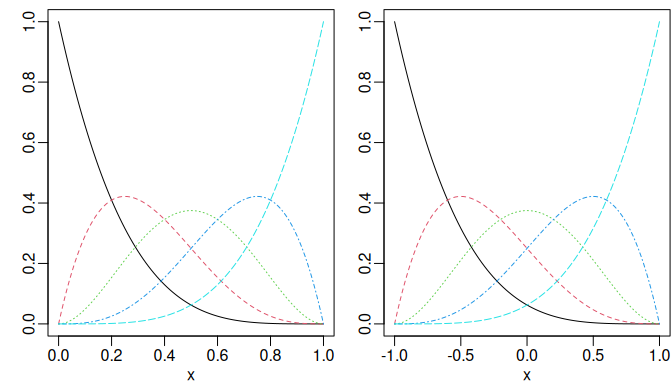Bernstein polynomials of degree 4 over [0, 1] (left) and the generalized version over [- 1, 1] (right).

In addition, we may specify integral = TRUE or derivs = 1 in bernsteinPoly() for their integrals or first derivatives, respectively.

ibpMat1 <- bernsteinPoly(x1, degree = 4, intercept = TRUE, integral = TRUE)
ibpMat2 <- bernsteinPoly(x2, degree = 4, intercept = TRUE, integral = TRUE)
dbpMat1 <- bernsteinPoly(x1, degree = 4, intercept = TRUE, derivs = 1)
dbpMat2 <- bernsteinPoly(x2, degree = 4, intercept = TRUE, derivs = 1)
par(mfrow = c(2, 2))
plot(ibpMat1, ylab = "Integrals")
plot(ibpMat2, ylab = "Integrals")
plot(dbpMat1, ylab = "Derivatives")
plot(dbpMat2, ylab = "Derivatives")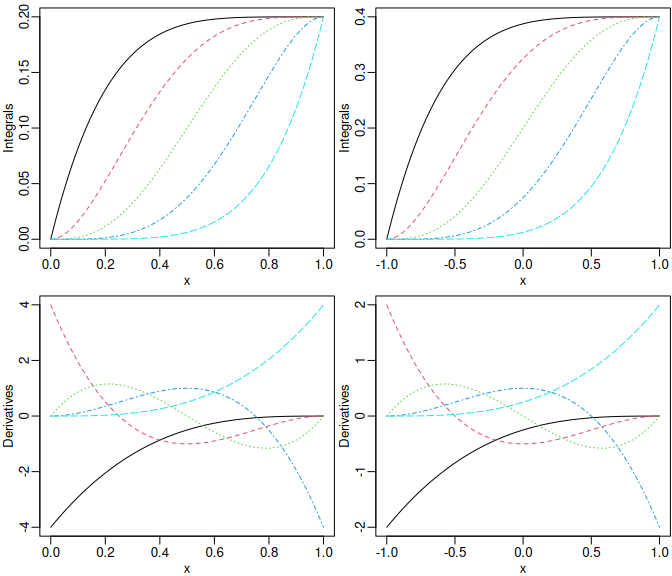The integrals (upper panel) and the first derivatives (lower panel) of Bernstein polynomials of degree 4.

Similarly, we may also use the deriv() method to get derivatives of an existing bernsteinPoly object.

stopifnot(is_equivalent(dbpMat1, deriv(bpMat1)))
stopifnot(is_equivalent(dbpMat2, deriv(bpMat2)))
stopifnot(is_equivalent(dbpMat1, deriv(ibpMat1, 2)))
stopifnot(is_equivalent(dbpMat2, deriv(ibpMat2, 2)))

# 7 Natural Cubic Splines

## 7.1 Nonnegative Natural Cubic Basis Functions

The package provides two variants of the natural cubic splines that can be constructed by naturalSpline() and nsk(), respectively, both of which are different from splines::ns().

The naturalSpline() function returns nonnegative basis functions (within the boundary) for natural cubic splines by utilizing a closed-form null space derived from the second derivatives of cubic B-splines. When integral = TRUE, the function naturalSpline() returns the integral of each natural spline basis.

nsMat <- naturalSpline(x, knots = knots, intercept = TRUE)
insMat <- naturalSpline(x, knots = knots, intercept = TRUE, integral = TRUE)
par(mfrow = c(1, 2))
plot(nsMat, ylab = "Basis")
plot(insMat, ylab = "Integrals")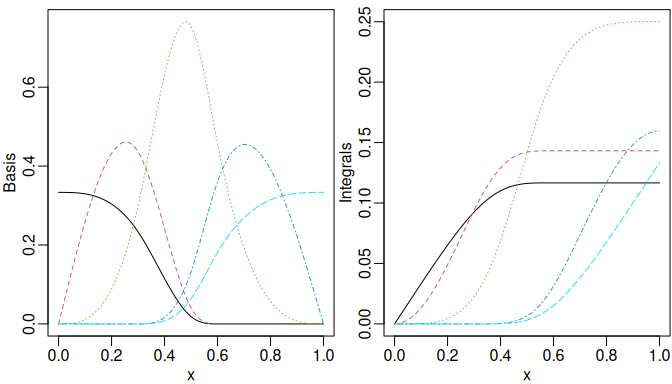Nonnegative natural cubic splines (left) and corresponding integrals (right).
stopifnot(is_equivalent(nsMat, deriv(insMat)))

Similarly, one may directly specify the argument derivs in naturalSpline() or use the corresponding deriv() method to obtain the derivatives of spline basis functions.

d1nsMat <- naturalSpline(x, knots = knots, intercept = TRUE, derivs = 1)
d2nsMat <- deriv(nsMat, 2)
matplot(x, d1nsMat, type = "l", ylab = "The 1st derivatives")
matplot(x, d2nsMat, type = "l", ylab = "The 2nd derivatives")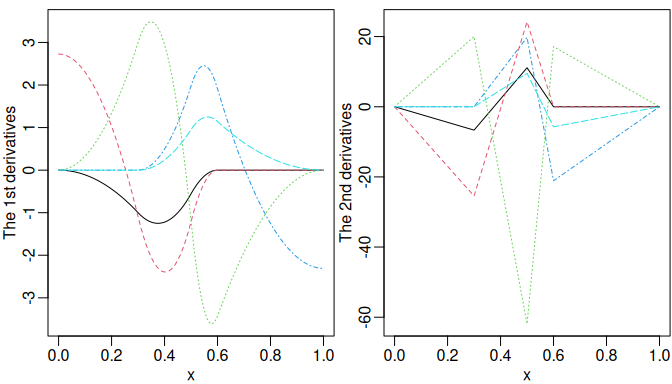The derivatives of natural cubic splines.

## 7.2 Natural Cubic Basis Functions with Unit Heights at Knots

The function nsk() produces another variant of natural cubic splines, where only one of the spline basis functions is nonzero with unit height at every boundary and internal knot. As a result, the coefficients of the basis functions are the values of the spline function at the knots, which makes it more straightforward to interpret the coefficient estimates. This idea originated from the function nsk() of the survival package (introduced in version 3.2-8). The implementation of the nsk() of splines2 essentially follows the survival::nsk() function. One noticeable argument for nsk() is trim (equivalent to the argument b of survival::nsk()). One may specify trim = 0.05 to exclude 5% of the data from both sides when setting the boundary knots, which can be a more sensible choice in practice due to possible outliers. The trim argument is also available for naturalSpline(), which however is zero by default for backward compatibility. An illustration of the basis functions generated by nsk() is as follows:

nskMat <- nsk(x, knots = knots, intercept = TRUE)
par(op)
plot(nskMat, ylab = "nsk()", mark_knots = "all")
abline(h = 1, col = "red", lty = 3)